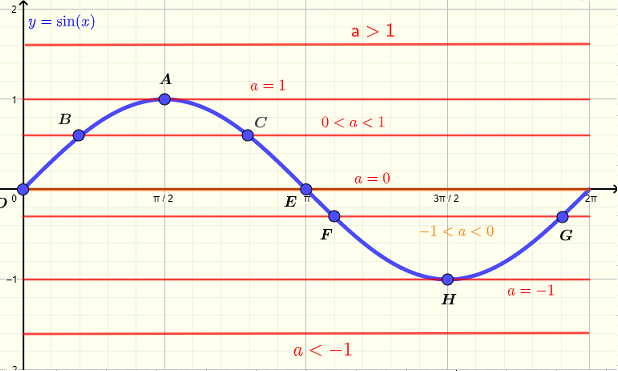# Sine Equation Solver

 

An online solver calculator for simple sine equations of the form $\sin x = a$ is presented.
Solution on the interval $[ 0, 2\pi)$ or $[ 0, 360^{\circ})$ are presented. (Note that the interval is open at $2\pi$ ).

## Graphical interpretations of the solutions of the equation $\sin(x)= a$ over the interval $[ 0, 2\pi)$

Below is shown the graph of $y = \sin(x)$ and $y = a$ (horizontal line in red) and it can be seen that for $a \gt 1$ or $a \lt - 1$, there are no points of intersection of the two graphs and therefore the equation $\sin(x) = a$ has no solution.
For $a = 1$, there is one point of tangency $A$ hence one solution given by $x = \dfrac{\pi}{2}$
For $a = - 1$, there is one point of tangency $H$ hence one solution given by $x = \dfrac{3\pi}{2}$
For all the remaining values of $a$, there are two points of intersections hence there are two solutions.
The calculator presented below allows to practice different cases as described above and hence fully understand how to solve equations of the form $\sin(x) = a$.## Analytical Solutions of the equation $\sin(x)= a$ over the interval $[ 0, 2\pi)$

1) The range of $y = \sin(x)$ is given by the interval $[ 1, -1]$ (see graph above). Therefore $\sin(x)$ cannot take values outside the interval $[ 1, -1]$ and therefore the equation $\sin(x) = a$ has no real solution for $a \gt 1$ or $a \lt -1$.
2) The solution to the equation $\sin(x) = 1$ is given by $x = \dfrac{\pi}{2}$
3) The solution to the equation $\sin(x) = -1$ is given by $x = \dfrac{3\pi}{2}$
4) The solutions to the equation $\sin(x) = 0$ are given by: $x_1 = 0$ and $x_2 = \pi$

5) For all other values of $a$, we solve the equation $\sin(x) = a$ as follows:
Let the reference angle $x_r = \arcsin(|a|)$
If $0 \lt a \lt 1$ , there are two solutions given by: $x_1 = x_r$ and $x_2 = \pi - x_r$
If $-1 \lt a \lt 0$ , there are two solutions given by: $x_1 = x_r + \pi$ and $x_2 = 2\pi - x_r$

## Use the calculator to solve the equation $\sin(x)= a$ for $x$ over the interval $[ 0, 2\pi)$

Enter $a$ as a real number and press "enter". You may also set the "Number of Decimal Places" desired as a positive integer.

 $a$ = 0.5 Number of Decimal Places = 3